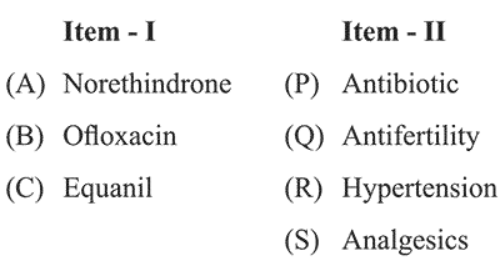# The correct match between item (I) and item (II) is :Question:

The correct match between item (I) and item (II) is :1. $(\mathrm{A}) \rightarrow(\mathrm{Q}) ;(\mathrm{B}) \rightarrow(\mathrm{R}) ;(\mathrm{C}) \rightarrow(\mathrm{S})$

2. $(\mathrm{A}) \rightarrow(\mathrm{Q}) ;(\mathrm{B}) \rightarrow(\mathrm{P}) ;(\mathrm{C}) \rightarrow(\mathrm{R})$

3. $(\mathrm{A}) \rightarrow(\mathrm{R}) ;(\mathrm{B}) \rightarrow(\mathrm{P}) ;(\mathrm{C}) \rightarrow(\mathrm{S})$

4. $(\mathrm{A}) \rightarrow(\mathrm{R}) ;(\mathrm{B}) \rightarrow(\mathrm{P}) ;(\mathrm{C}) \rightarrow(\mathrm{R})$

Correct Option: , 2

Solution:

(A) Norethindrone - Antifertility (Q)

(B) Ofloxacin-Antibiotics (P)

(C) Equanil - used for hypertension (R)